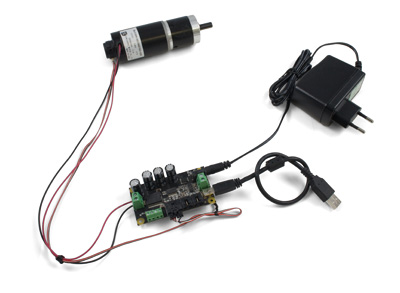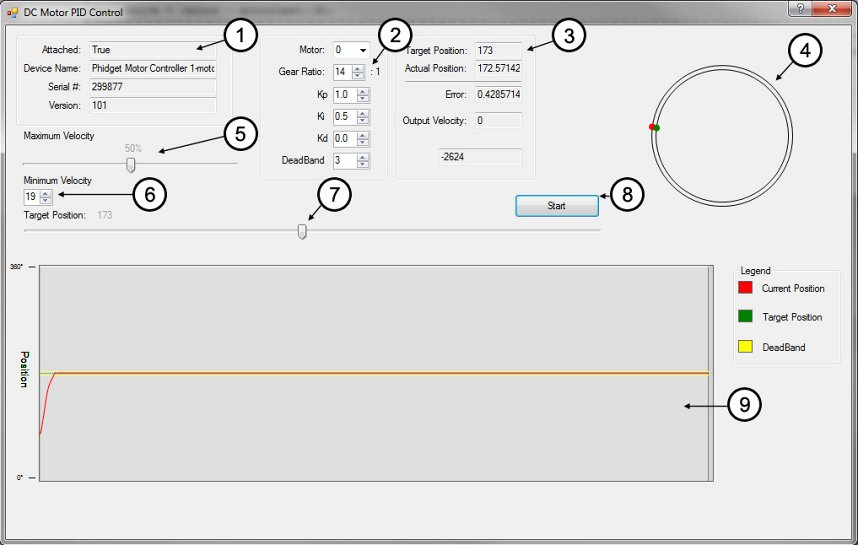Products for USB Sensing and ControlProducts for USB Sensing and Control#### sales inquiries

##### quotes, distributor information, purchase orders
sales@phidgets.com

#### technical inquiries

##### support, advice, warranty, returns, misshipment
support@phidgets.com

#### website inquiries

web@phidgets.com

##### Unit 1 - 6115 4 St SE Calgary AB  T2H 2H9 Canada
Projects Dealers Terms and Conditions Discontinued Products Phidget21 Documentation

# An application guide on DC Motors - PID Control

#### This guide explains the basics of PID control and how to implement a basic control loop using Phidgets

by MikeA DC motor is a motor that takes DC power in and converts it to rotational motion. Since DC motors can ordinarily only be told how fast to go (on a scale from -100% to 100% duty cycle) you need to program a control system. In order to make a control system, you'll need a motor that has a feedback device such as an encoder.

## Introduction

This application guide is designed to explain the basics of PID control and how to implement a basic control loop using Phidgets. Once implemented, the control loop will allow the DC motor to move to a precise position and remain there, even if an external force tries to move it.

## Phidgets

The following setup was used to build this application guide:

### PID Control Basics

The goal of a control loop is to use data from the feedback device (in this case, the encoder) to iteratively adjust the output until it has reached the target value.

For example, consider this simple control loop (This code would be used in the encoder change event handler):


DutyCycle = TargetPosition - ActualPosition;
if (DutyCycle > 100)
DutyCycle = 100;
if (DutyCycle < -100)
DutyCycle = -100;



Whenever the encoder value (ActualPosition) changes, the event handler triggers and updates the Duty Cycle of the motor. Eventually, this will result in the motor reaching its target. The following table further illustrates how this works:

Target Position Current Position Error Duty Cycle
180 0 180 100%
180 160 20 50%
180 170 10 25%
180 190 -10 -25%
180 180 0 0%

The type of control we've just implemented here is called Proportional control, because the output is directly proportional to the error. We can multiply (TargetPosition - ActualPosition) by a gain value if we want to control how the control loop behaves when it gets close to the target. A lower value (i.e. less than 1) will result in a more gradual reduction in speed as the motor reaches its target position, while a larger value will result in a sharper, quicker response. We'll call this value the proportional gain, or Kp for short. If the proportional gain is set too high, the motor will overshoot the target position and oscillate back and forth around the target.

While we've gained basic control of the motor position, there are still some problems with purely proportional control. You may find that the motor never quite reaches the target position, especially with a low proportional gain. Also, if the load on the motor changes while it's moving, it won't be able to react to the difference. To solve these problems, we need an integral term.

### Integral Control

The purpose of the integral term in a control loop is to look back at all of the past error and accumulate it into an offset that the control loop can use. Combined with our proportional control loop, it will be able to react to changes in load and land closer to the target position if we choose our gain values properly.


dt = 0.008;
error = TargetPosition - ActualPosition;
DutyCycle = (Kp * error) + (Ki * integral);

if (DutyCycle > 100)
DutyCycle = 100;
else if (DutyCycle < -100)
DutyCycle = -100;
else
integral += (error * dt);



Where dt is the change in time between iterations of the control loop. For this example, dt is set to 0.008 seconds, which is the update rate of the encoder event. The integral is only accumulated when the duty cycle isn't saturated at 100% or -100%. This prevents the control loop from needlessly accumulating the integral when it's already running at maximum velocity. Now we have two control gains: Kp and Kp still functions as it did in the proportional example, and Ki controls how influential the integral term is. Increasing the integral gain will cause the control loop to reach the target faster, and ensure that you hit the exact target rather than settling to a value close to the target. Just like the proportional gain, increasing the integral gain too much can cause instability.

After tweaking the control gains, you may find that PI control is sufficient for your application. If you're having trouble finding control gains that provide the results you're looking for, you can implement full PID control by adding the derivative term.

### Derivative Control

Derivative control looks at past errors in the system and calculates the slope of those errors to predict future error values. Adding derivative control should add stability to the system and increase the control we have over it by adding another gain value.


dt = 0.008;
error = TargetPosition - ActualPosition;
DutyCycle = (Kp * error) + (Ki * integral) + (Kd * derivative);

if (DutyCycle > 100)
DutyCycle = 100;
else if (DutyCycle < -100)
DutyCycle = -100;
else
integral += (error * dt);

derivative = (error - errorlast)/dt;



Where errorlast is the error value from a set number of samples ago. In an application such as this, an error value from around 64 samples ago (0.5 seconds) should suffice. Just as before, we can now use Kd to modify the weight of this new derivative term. Increasing Kd should smooth things out and increase stability, but you'll find that it should be kept small in comparison to the other control gains or it will cause the control to oscillate when it's near the target.

## Sample Program

You can download the sample program written for this guide. This is a Visual C# project.

Note: The code examples in this article use Phidget21 code and may not work with newer versions of the Phidget library.

### Controls And Settings1. These boxes contain the information of the attached motor controller. If these boxes are blank, it means the controller object didn't successfully attach.
2. These boxes control which motor output is being used, and allow you to set each of the control gains. You can also enter the gearbox ratio so that the position slider will map to a full 360 degree rotation on your motor. You can click on the "?" icon in the top right for more information on a particular setting. Changes will not take effect until the "Start" button is hit.
3. These boxes display the target position set by the slider and the actual position according to the feedback encoder. The error is the difference between these two values, and the output velocity is the duty cycle of the controller as dictated by the control loop.
4. This graph is a visual representation of the current position (red) relative to the target position (green).
5. The maximum velocity slider controls the maximum duty cycle that the controller will output. It is unrestricted (100%) by default.
6. The minimum velocity box exists because some gear motors require a minimum amount of torque just to move the shaft even with no load. To determine what to set the minimum velocity to, enter the control panel and slowly turn up the speed. As soon at the motor actually moves, that number is a suitable minimum velocity.
7. This slider selects the target position, from 1 to 360° (where 1° corresponds to the starting position of the motor shaft when the program is started).
8. Pressing the start button will initiate the control loop and the motor will begin to move toward the target position. If the motor does not move, check your power and connections.
9. This graph will plot the motor's position and the target position over time.

### Algorithm

The PID control algorithm in this sample program operates by setting up a change handler for the encoder, which then calls an external function that computes the PID control loop output as briefly described in the previous section. By changing the output, the motor's duty cycle is set and the function will be called again the next time the encoder change handler triggers (in 0.008 seconds, because the sampling period of an encoder input is 8ms). This loop will continue until the output of the control loop is zero and the motor has reached its target.


// This event handler fires every 8ms, regardless of whether the position has changed.
// If you want to create an event that only fires when position is changed, use "EncoderPositionChange"

void dcMotor_PositionUpdate(object sender, EncoderPositionUpdateEventArgs e)
{
// Divide the encoder value by the gearbox reduction ratio, since the encoder is on
// the rear shaft and rotates much faster than the primary shaft
// Then mod 360 since this program only controls the position of the motor
// and not the number of rotations
feedback = (double)(dcMotor.encoders.Position / gearTxt.Value) % 360;
trueEncoderTxt.Text = dcMotor.encoders.Position.ToString();

// If feedback is negative, loop back around to 360
if (feedback < 0)
{
feedback += 360;
}

// If the start button has been pressed,
if (started)
{
// Calculate and set the new output from the control loop
PID();
dcMotor.motors[Convert.ToInt32(motorCmb.Text)].Velocity = Math.Round(output);
}
}



It's always a good idea to keep your event handlers as small and optimized as possible to avoid missing events. In this sample program, all of the complexity of the PID algorithm is tucked away in the function PID().

The PID() function:

• Checks to see if the motor has reached its target (and if it has, stops the loop)
• Updates the the output (duty cycle) of the control loop
• Limits the duty cycle to the specified output limit

In the downloadable example, this function also updates the graph and all of the text boxes that track the variables. These lines were removed from the excerpt below for increased readability.


// This function does the control system calculations
// and sets output to the duty cycle that the motor needs to run at.

void PID()
{
// Calculate how far we are from the target
errorlast = error;
error = distance360(input, feedback);

// If the error is within the specified deadband, and the motor is moving slowly enough
// Or if the motor's target is a physical limit and that limit is hit
if ((Math.Abs(error) <= deadBand && Math.Abs(output) <= (double)vminTxt.Value))
{
// Stop the motor
output = 0;
error = 0;
}
else
{
// Else, update motor duty cycle with the newest output value
// This equation is a simple PID control loop
output = ((Kp * error) + (Ki * integral)) + (Kd * derivative);

//output = Kp * error;

errorTxt.Text = error.ToString();
}

// Prevent output value from exceeding maximum output specified by user,
// And prevent the duty cycle from falling below the minimum velocity (excluding zero)
// The minimum velocity exists because some DC motors with gearboxes will not be able
// to overcome the detent torque of the gearbox at low velocities.
if (output >= maxOutput)
output = maxOutput;
else if (output <= -maxOutput)
output = -maxOutput;
else if (output < (double)vminTxt.Value && output > 0)
output = (double)vminTxt.Value;
else if (output > ((double)vminTxt.Value)*(-1) && output < 0)
output = ((double)vminTxt.Value)*(-1);
else
integral += (error * dt);

// Calculate the derivative for the next iteration
derivative = (error - errorlast) / dt;

// Record the previous encoder value for the next iteration of the control loop
feedbacklast = feedback;

}